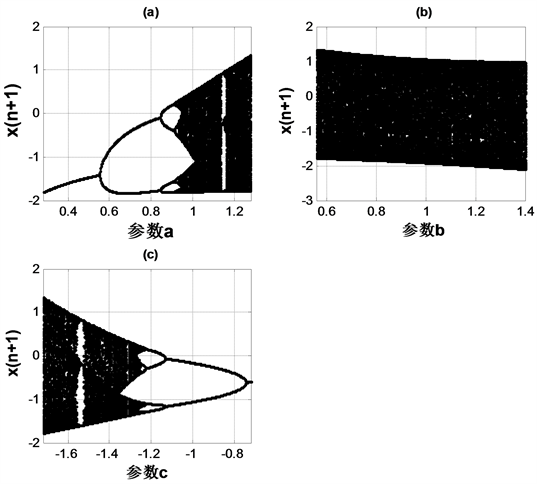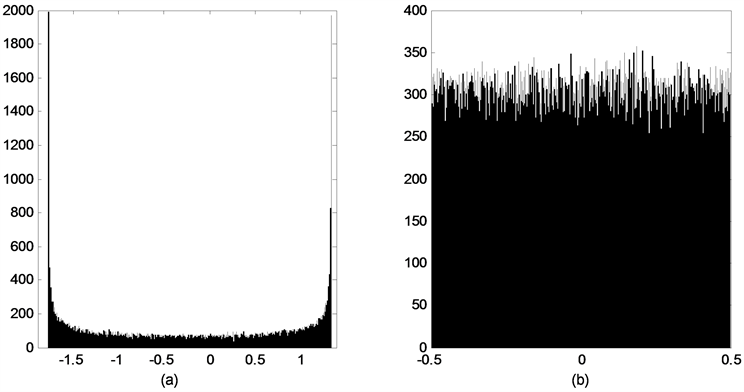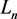﻿ 基于均匀化混沌系统的S-Box生成算法

基于均匀化混沌系统的S-Box生成算法Algorithm of Generating S-Box Based on Uniform Chaotic System

Abstract: In this paper, a new quadratic polynomial chaotic system is given and homogenized based on its probability density function. Then, based on the homogenized chaotic systems, an S-box generation algorithm is constructed. The security of S-box is tested by numerical simulation including differential probability analysis and linear probability analysis. The statistical results show that the uniform chaotic system can produce better performance of S-boxes.

1. 引言

2. 二次多项式混沌系统的均匀化处理

${b}^{2}-4ac-2b\ge 7$

$f\left(x\right)=1.28{x}^{2}+0.56x-1.72,x\in \left[-\frac{57}{32},\frac{43}{32}\right]$ (1)

$x\left(n+1\right)=a{x}^{2}\left(n\right)+bx\left(n\right)+c$ (2)

$a=1.28,b=0.56,c=-1.72.$

$f\left(x\right)=1.28{x}^{2}+0.56x-1.72,x\in \left[-\frac{57}{32},\frac{43}{32}\right]$

$g\left(x\right)=\left\{\begin{array}{l}2x,\text{}0\le x\le \frac{1}{2}\\ 2-2x,\text{}\frac{1}{2}\le x\le 1\end{array}$Figure 1. (a) The bifurcation diagram of parameter a in system (2); (b) The bifurcation diagram of parameter b in system (2); (c) The bifurcation diagram of parameter c in system (2)

$h\left(x\right)=\frac{25}{16}\mathrm{cos}\text{π}x-\frac{7}{32},\text{\hspace{0.17em}}x\in \left[0,1\right]$

$\begin{array}{c}h\circ g\left(x\right)=\left\{\begin{array}{l}\frac{25}{16}\mathrm{cos}2\text{π}x-\frac{7}{32},\text{\hspace{0.17em}}\text{\hspace{0.17em}}\text{\hspace{0.17em}}\text{\hspace{0.17em}}\text{\hspace{0.17em}}\text{\hspace{0.17em}}\text{\hspace{0.17em}}\text{\hspace{0.17em}}\text{\hspace{0.17em}}\text{\hspace{0.17em}}\text{\hspace{0.17em}}\text{\hspace{0.17em}}\text{\hspace{0.17em}}0\le x\le \frac{1}{2}\\ \frac{25}{16}\mathrm{cos}\text{π}\left(2-2x\right)-\frac{7}{32},\text{\hspace{0.17em}}\text{\hspace{0.17em}}\text{\hspace{0.17em}}\text{ }\text{\hspace{0.17em}}\frac{1}{2}\le x\le 1\end{array}\\ =\frac{25}{16}\mathrm{cos}2\text{π}x-\frac{7}{32}\\ =h\left(2x\right)\end{array}$

$\begin{array}{c}f\circ h\left(x\right)=\frac{32}{25}{h}^{2}\left(x\right)+\frac{14}{25}h\left(x\right)-\frac{43}{25}\\ =\frac{32}{25}{\left(h\left(x\right)+\frac{7}{32}\right)}^{2}-\frac{57}{32}\\ =\frac{32}{25}{\left(\frac{25}{16}\mathrm{cos}\text{π}x\right)}^{2}-\frac{57}{32}\\ =\frac{25}{16}\mathrm{cos}2\text{π}x-\frac{7}{32}\\ =h\left(2x\right)\end{array}$

$h\left(g\left(x\right)\right)=f\left(h\left(x\right)\right)$$f\left(x\right)$ 与Tent映射关于 $h\left(x\right)$ 拓扑共轭。

$f\left(x\right)=1.28{x}^{2}+0.56x-1.72,\text{\hspace{0.17em}}x\in \left[-\frac{57}{32},\frac{43}{32}\right]$

$\rho \left(x\right)=\left\{\begin{array}{l}\frac{1}{\text{π}}\frac{32}{\sqrt{2451-448x-1024{x}^{2}}}\text{,}x\in \left[-\frac{57}{32},\frac{43}{32}\right]\\ 0,\text{}其他\end{array}$

${\rho }_{T}\left(x\right)=1,\text{\hspace{0.17em}}x\in \left(0,1\right).$

$\begin{array}{c}{\rho }_{f}\left(x\right)={\rho }_{T}\left({h}^{-1}\left(x\right)\right)|\frac{\text{d}{h}^{-1}\left(x\right)}{\text{d}x}|\\ =\frac{1}{\text{π}}\frac{32}{\sqrt{2451-448x-1024{x}^{2}}}\end{array}$

$\rho \left(x\right)=\left\{\begin{array}{l}\frac{1}{\text{π}}\frac{32}{\sqrt{2451-448x-1024{x}^{2}}}\text{,}x\in \left[-\frac{57}{32},\frac{43}{32}\right]\\ 0,\text{}其他\end{array}$

$Z=\frac{1}{\text{π}}\mathrm{arcsin}\left(-\frac{16}{25}X-\frac{7}{50}\right)$ (3)

$\begin{array}{c}{F}_{Z}\left(z\right)=P\left(Z\le z\right)=P\left(\frac{1}{\text{π}}\mathrm{arcsin}\left(-\frac{16}{25}X-\frac{7}{50}\right)\le z\right)\\ ={\int }_{-\infty }^{-\frac{25}{16}\mathrm{sin}\text{π}z-\frac{7}{32}}{\rho }_{X}\left(x\right)\text{d}x\end{array}$ (4)

${\rho }_{Z}\left(z\right)=\left\{\begin{array}{c}1,\text{}-\frac{1}{2}\le z\le \frac{1}{2};\\ 0,\text{}\text{ }其它.\text{}\end{array}$

$\left\{\begin{array}{l}x\left(n+1\right)=1.28x{\left(n\right)}^{2}+0.56x\left(n\right)-1.72\\ z\left(n+1\right)=\frac{1}{\text{π}}\mathrm{arcsin}\left(-\frac{16}{25}x\left(n+1\right)-\frac{7}{50}\right)\end{array}$ (5)

3. 基于均匀化混沌系统构造S-Box

3.1. S-Box算法构造

S-Box作为分组密码中的非线性部件，主要起到置换的功能。为了达到更好的置乱效果，增加加密方案的抵抗攻击能力，本文利用混沌系统进行迭代，并基于混沌系统的不同的初始值动态生成 $8×8$ 的S-Box，因而，S-Box的密钥由混沌系统的参数，迭代次数，初值等构成。具体的算法步骤为：Figure 2. Histogram: (a) Histogram of the sequence before uniformity; (b) Histogram of the sequence after uniformity

1) 将相空间 $\left[-0.5,0.5\right]$ 进行n等分，分别为每个小区间标号为 $i=0,1,\cdots ,n$

2) 取初值 ${x}_{0}$ ，利用混沌系统(5)式迭代n次，得到一个n维混沌序列；

3) 将序列值对应到相空间 $\left[-0.5,0.5\right]$ 中的相应小区间，用区间标号 $i\left(\mathrm{mod}256\right)$ ，得到一个n维位置序列；

4) 位置序列中前256个不相等的值依次构成S-Box序列。

3.2. S-Box性能分析

 ，线性运算之和都为128，与理想值相同，满足双射特性，输

S-Box的非线性度为 ${N}_{s}=\underset{\begin{array}{l}\text{\hspace{0.17em}}\text{\hspace{0.17em}}\text{\hspace{0.17em}}l\in {L}_{n}\\ 0\ne u\in {F}_{2}^{m}\end{array}}{\mathrm{min}}{d}_{H}\left(u\cdot S\left(x\right),l\left(x\right)\right)$ 。式中： $u\cdot S\left(x\right)$ 表示u与 $S\left(x\right)$ 的点积。表示全体n元线性和仿射函数之集； ${d}_{H}\left(x,y\right)$ 表示x和y的汉明距离。函数的非线性度越大，意味着抵抗线性

S-Box的差分概率(DP)用来评价其抵抗差分密码攻击的能力，DP值越小，抵抗能力越强。而线性概率(LP)评价了S-box抵抗线性密码攻击的能力，LP 指标越小，抵抗效果越好。离散混沌S-Box的Lyapunov指数  可以用来衡量双射映射中自变量改变一比特时，映射值变化比特的情况。Lyapunov指数越大，说明自变量能够引起状态值的变成程度越高，意味着系统混沌性越好。本文构造S-Box的DP，LP及其Lyapunov指数结果与文献中S-Box的对比见表3。由对比结果可见，本文S-box的DP值和LP值均小于Table 2. Comparison of the nonlinearity of S-boxesTable 3. Comparison of the DP, LP, Lyapunov exponent of S-boxes

4. 结论

 Li, Tienyien. and Yorke, J.A. (1975) Period Three Implies Chaos. American Mathematical Monthly, 82, 985-992.
https://doi.org/10.1080/00029890.1975.11994008

 Matthews, R. (1989) On the Serivation of a “Chaotic” Encryption Algorithm. Cryptologia, 13, 29-42.
https://doi.org/10.1080/0161-118991863745

 Gotz, M., Kelber, K. and Schwarz, W. (1997) Discrete-Time Chaotic Coders for Information Encryption—Part 1: Systematic Structural Design. Workshop on Nonlinear Dynamics of Electronic Systems, Moscow, Russia, 21-26.

 Kocarev, L., Jakimoski, G., Stojanovski, T., et al. (1998) From Chaotic Maps to Encryption Schemes. Proceedings of the 1998 IEEE International Symposium on Circuits and Systems, Monterey, CA, USA, 31 May-3 June 1998, 514-517.
https://doi.org/10.1109/ISCAS.1998.698968

 盛利元, 曹莉凌, 孙克辉, 等. 基于TD-ERCS混沌系统的伪随机数发生器及其统计特性分析[J]. 物理学报, 2005, 54(9): 4031-4037.

 盛利元, 肖燕予, 盛喆. 将混沌序列变换成均匀伪随机序列的普适算法[J]. 物理学报, 2008, 57(7): 4007-4013.

 曹光辉, 胡凯, 佟维. 基于Logistic均匀分布图像置乱方法[J]. 物理学报, 2011, 60(11): 125-132.

 何振亚, 李克, 杨绿溪. 具有良好安全性能的混沌映射二进制序列[J]. 电子与信息学报, 1999, 21(5): 646-651.

 Terry, R. (1990) Substitution Cipher with Pseudo-Random Shuffling: The Dynamic Substitution Combiner. Cryptologia, 14, 289-303.
https://doi.org/10.1080/0161-119091864986

 Wong, K.W., Ho, S.W. and Yung, C.K. (2003) A Chaotic Cryptography Scheme for Generating Short Cipher Text. Physics Letters A, 310, 67-73.
https://doi.org/10.1016/S0375-9601(03)00259-7

 周海玲, 宋恩彬. 二次多项式映射的3-周期点判定[J]. 四川大学学报(自然科学版), 2009, 46(3): 561-564.

 郝柏林. 从抛物线谈起—混沌动力学引论[M]. 北京: 北京大学出版社, 2013: 114-118.

 臧鸿雁, 范修斌, 闵乐泉, 等. S-Box的Lyapunov指数研究[J]. 物理学报, 2012, 61(20): 200508.

 Han, M., Shah, T. and Batool, S.I. (2016) Construction of S-Box Based on Chaotic Boolean Functions and Its Application in Image Encryption. Neural Computing & Applications, 27, 677-685.
https://doi.org/10.1007/s00521-015-1887-y

 韩丹丹, 闵乐泉, 赵耿, 等. 一维鲁棒混沌映射及S-Box的设计[J]. 电子学报, 2015(9): 1770-1775.

 Razaq, A., Yousaf, A., Shuaib, U., et al. (2017) A Novel Construction of Substitution Box Involving Coset Diagram and a Bijective Map. Security & Communication Networks, 2017, Article ID: 5101934.
https://doi.org/10.1155/2017/5101934

Top